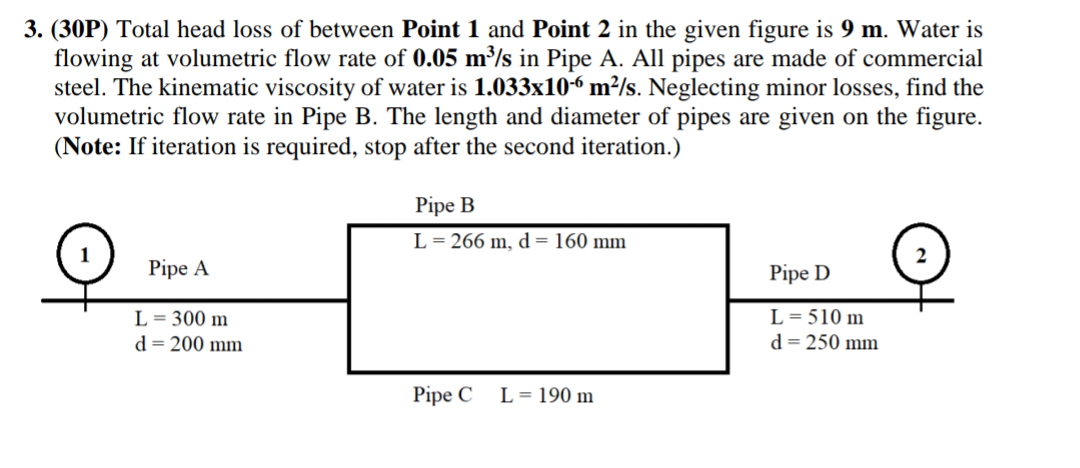# Question Solved1 AnswerTotal head loss ofbetween Point 1 and Point 2 in the given figure is 9 m. Water isflowing at volumetric flow rate of 0.05 m3/s in Pipe A. Allpipes are made of commercial steel. The kinematic viscosity ofwater is 1.033x10-6 m2/s. Neglecting minor losses, findthe volumetric flow rate in Pipe B. The length and diameter ofpipes are given on the figure. (Note: If iteration isrequired, stop after the second iteration.) 3. (30P) Total head loss of between Point 1 and Point 2 in the given figure is 9 m. Water is flowing at volumetric flow rate of 0.05 m°/s in Pipe A. All pipes are made of commercial steel. The kinematic viscosity of water is 1.033x10-6 m²/s. Neglecting minor losses, find the volumetric flow rate in Pipe B. The length and diameter of pipes are given on the figure. (Note: If iteration is required, stop after the second iteration.) Pipe B L = 266 m, d = 160 mm Pipe A Pipe D L = 300 m d=200 mm L = 510 m d=250 mm Pipe C L = 190 mPN9TUY The Asker · Mechanical EngineeringTotal head loss of between Point 1 and Point 2 in the given figure is 9 m. Water is flowing at volumetric flow rate of 0.05 m3/s in Pipe A. All pipes are made of commercial steel. The kinematic viscosity of water is 1.033x10-6 m2/s. Neglecting minor losses, find the volumetric flow rate in Pipe B. The length and diameter of pipes are given on the figure. (Note: If iteration is required, stop after the second iteration.)

Transcribed Image Text: 3. (30P) Total head loss of between Point 1 and Point 2 in the given figure is 9 m. Water is flowing at volumetric flow rate of 0.05 m°/s in Pipe A. All pipes are made of commercial steel. The kinematic viscosity of water is 1.033x10-6 m²/s. Neglecting minor losses, find the volumetric flow rate in Pipe B. The length and diameter of pipes are given on the figure. (Note: If iteration is required, stop after the second iteration.) Pipe B L = 266 m, d = 160 mm Pipe A Pipe D L = 300 m d=200 mm L = 510 m d=250 mm Pipe C L = 190 m
More
Transcribed Image Text: 3. (30P) Total head loss of between Point 1 and Point 2 in the given figure is 9 m. Water is flowing at volumetric flow rate of 0.05 m°/s in Pipe A. All pipes are made of commercial steel. The kinematic viscosity of water is 1.033x10-6 m²/s. Neglecting minor losses, find the volumetric flow rate in Pipe B. The length and diameter of pipes are given on the figure. (Note: If iteration is required, stop after the second iteration.) Pipe B L = 266 m, d = 160 mm Pipe A Pipe D L = 300 m d=200 mm L = 510 m d=250 mm Pipe C L = 190 m